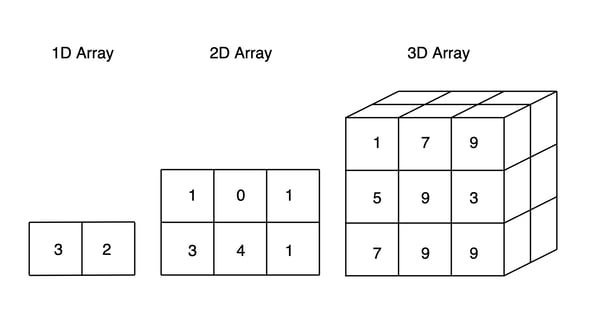Related Tags

arrays
3d arrays
3d arrays java
3d arrays c++

# What is a 3-D array?Educative Answers Team

Arrays are used to store data and information on various data types. The greatest advantage of arrays is that they allow all data to be accessed in O(1) time complexity.

## 3-D arrays

3-D arrays are referred to as multi-dimensional arrays. Multi-dimensional arrays are defined as an “array of arrays” that store data in a tabular form.

Imagine this, an array list of data elements makes a 1-D (one-dimensional) array. An array of 1-D arrays makes a 2-D (two-dimensional) array. Similarly, an array of 2-D arrays makes a 3-D ( three-dimensional) array.

A 3-D array can be declared as follows:

int arr
// declares an 3-D integer array


This array can store a total of 4 X 5 X 8 = 160 elements.An illustration to understand concept of 3D array

## Code

#include <iostream>
using namespace std;

int main() {
int arr =
{
{ {0,1,2}, {2,3,4}, {6,7,1} },
{ {6,7, 1}, {8,9, 2}, {9,14, 22} }
};

// accessing a single value i.e 0
cout << arr << endl;

// output each element's value by iterating through the array
for (int i = 0; i < 2; ++i)
{
for (int j = 0; j < 3; ++j)
{
for (int k = 0; k < 3; ++k)
{
cout << "Element at arr[" << i << "][" << j
<< "][" << k << "] = " << arr[i][j][k]
<< endl;

}
}
}
return 0;
}


RELATED TAGS

arrays
3d arrays
3d arrays java
3d arrays c++